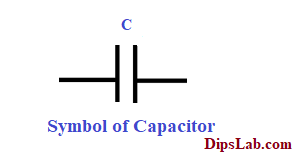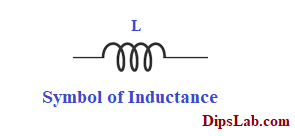# 12 Difference between Capacitor and Inductor | Similarities, DefinitionIn this tutorial, we are going to study about what is difference between capacitor and inductor with their different specifications.  We will also cover the similarities between them.

### Definition of Capacitor and Inductor

#### What is the Capacitor?

The device that produces or possesses capacitance property is called as a Capacitor.

Sometimes the capacitor called a Condenser.

#### What is the Inductor?

The device that produces or possesses inductance property is called as an Inductor.

Sometimes the inductor called as Coil or Choke.

Let’s dive into the basic difference between Capacitance and Inductance in tabular format.

### Difference between Capacitor and Inductor

 Sr. No. Content Capacitance Inductance 01 Definition of capacitance & Inductance The property of the device that stores electrical energy in the form of electric charges is called as Capacitance. The property of the device that stores magnetic energy in the form of magnetic lines or fluxes is called as Inductance. 02 Signified Capacitance is denoted by the capital letter ‘C’. Inductance is denoted by the capital letter ‘L’. 03 Unit of capacitor & inductor The unit of the Capacitance is Farad (F). The unit for inductance is the Henry (H). 04 Working Role The capacitor is the circuit element in which the energy is stored in an Electrostatic form. The inductor is the circuit element in which the energy is stored in an Electromagnetic form. 05 What is the main function of inductor & capacitor? The main function of capacitance is to oppose the change in voltage. The main function of inductance is to oppose the change in electric current. 06 Energy Stored of  Capacitor & Inductor The capacitor is capable of storing electrical energy in the form of capacitance. The total energy stored in the capacitor can be calculated as – `W = [(1/2)*C*V²]` An inductor is capable of storing magnetic energy in the form of inductance. The total energy stored in the inductor can be calculated as – `W = [(1/2)*L*I²]` 07 Types of capacitor & inductor Capacitors are classified into different types -Ceramic, Tantalum, and Electrolytic. Inductors are classified into different types – Core, Coupled, Molded. 08 Power Factor of capacitor & inductor In a capacitive circuit, the power factor is said to be leading, if the current leads the voltage. In an Inductive circuit, the power factor is said to be lagging if the current lags behind the voltage. 09 Insulation (Insulating Materials) Insulation is provided over the conductor. Insulation does not provide over the conductor. 10 What is the application of inductor & capacitor? The capacitor is used in electrical and electronic circuits or devices, power factor correction, etc. The inductor is used in domestic applications,  transmission and distribustion systems, analog circuits, and signal processing, etc.

#### 11. Symbolic Representation of Capacitor and Inductor

•  Capacitance / Capacitor Symbol:

The symbol of conductance is represented by the two conducting plates with inserting dielectric (insulating) materials in between the two plates.•  Inductance / Inductor Symbol:

The symbol of inductance is represented by the winding (or turns) of the coil on the magnetic core.#### 12. Capacitor and inductor formula

Calculating the value capacitance and inductance by using basic formula-

• Capacitance Calculator
`Capacitance, C = (Q/V)     (Unit- Farad)`

Where

Q- Charge particle (Unit- Coulomb)

V- Potential difference between the capacitor (Unit – Volt)

Or

`Capacitance, C = (ε*A/d)     (Unit- Farad)`

A- Area between the conductor (Unit- Meter²)

d- Difference between two conducting plates (Unit- Meter)

ε- Permittivity of an electrical field (Unit – Farad/Meter )

You can calculate the value of the capacitor by using online tools.

• Inductance Calculator
`Inductance, L = (∅/MMF)     (Unit-Henry)`

Where,

∅- Number of flux generating in the magnetic field (Unit – Weber)

MMF- Magneticmotive Force developed between the magnetic circuit. (Unit -Ampere-turn)

You can calculate the value of the inductor by using online tools.

### Similarities between Capacitor and Inductor

Here is listing the similarity between capacitance and inductance.

• The circuit elements of capacitance and inductance are used to store the generated energy.
• These two circuit elements are used in alternating current (AC) circuits.
• Capacitor and Inductor are passive elements. It means they don’t generate energy by themself. (You can read more about active and passive elements in the circuit.)
• Both capacitors and inductors are used in the LC Filter or Π Filter circuit.

So, I have covered 12 different points of inductor vs capacitor and also similarities between them.

If you have any queries regarding capacitors and capacitance vs. inductors and inductance, you can ask in the given comment.

Related differences you should learn:

Practice Now »

### 22 thoughts on “12 Difference between Capacitor and Inductor | Similarities, Definition”

1.•Thanks, Yagnesh:)

2.Very good Content!

•Thanks, Henry 🙂

•Thanks, Henry:)

3.Nice work Dipali.

4.Hello, I’m in the US. It is not very common to find such a range of informative topics for free. Thank you very much.

•You’re welcome, Chaunlaw.

And thanks for reaching out to my blog. I am promising that, I will share more informative technical tutorials.

5.Very useful content.

Thank you mam!

•You’re welcome, Dear:)

6.Differences :
Inductor : stores in electromagnetic form energy, if arranged in series or parallel both of them have difference ways to calculate. The higher of frequency, the higher of its value and vice versa with the capacitor.
The unit in Henry.

Capacitor : stores in electric field energy.

Similarities :
Both of them are impedances in electric circuit.
Both of them are calculated in Ohm.
Both of them reduce its values each other.
In RLC circuit, if L and C values are the same, then the impedance is only R value left.

•Thanks for sharing. I will add some points.

7.The capacitor is an isolated system. Inductor series-connected coil.

•Thanks, Manoj 🙂

8.Both are temporary storage element where,
Capacitor: stores energy in Electro-“static” form
Inductor: stores energy in Electro- “magnetic” form.

•Thanks, Nitish. I have already mentioned this point.

9.Almost key technical points are already mentioned above by experts.

Ideal Capacitor works short circuit when fully discharged and open switch when fully charge.. while inductor behaves fully reverse.

•10.From phasor point of view in case of capacitor current lead the voltage by 90° but in case of indicator voltage lead the current by 90° .
Capacitor is generally leading in nature and inductor is lagging in nature.

•Thanks, I forgot this point.

11.•